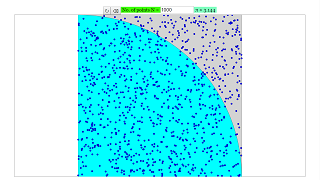About Estimation of PI using a Monte Carlo method:

Monte Carlo methods are often useful for solving problems in physics and mathematics, which cannot be solved by analytical means.
The goal of this virtual-lab is to obtain an approximated value of PI, using a Monte Carlo method. Next, we describe briefly how this method is used to estimate PI.
Imagine that we are throwing a dart over a square surface of side 1 m. There is a circle of radius 1 m. inscribed in the square. Assuming that the probability is uniformly distributed, the probability of hitting inside the circle is equal to PI/4. Dividing the number of hits by the number of throws, it is obtained an estimation of PI. We will obtain a better approximation by increasing the number of throws.
This virtual-lab allows the user to select the number of throws, with a maximum of 10000 throws. Author Carla Martín
Dpto. de Informática y Automática
E.T.S. de Ingeniería Informática, UNED
Juan del Rosal 16, 28040 Madrid, Spain

### Translations

Code Language Translator Run### Software Requirements

SoftwareRequirements

 Android iOS Windows MacOS with best with Chrome Chrome Chrome Chrome support full-screen? Yes. Chrome/Opera No. Firefox/ Samsung Internet Not yet Yes Yes cannot work on some mobile browser that don't understand JavaScript such as..... cannot work on Internet Explorer 9 and below

### CreditsCarla Martn; Tan Wei Chiong; Loo Kang Wee

[text]

### For Teachers

Before, we had a simulation on the calculation of π using Archimedes' algorithm. This simulation shows yet another method that can be used to approximate the value of π.

The Monte Carlo method is done by taking a unit square (a square of length 1) and inscribing a quadrant inside. A arbitrary number of points is then randomly scattered in the square. Since the area of the square is 1 and the area of the inscribed quadrant is π/4, the ratio of the number of points that land in the quadrant to the total number of points becomes an estimate of π/4, which is then multiplied by 4 to estimate π.

This method is used widely in mathematics and physics when a problem cannot be solved analytically.

The number of points can be set up to 50000 points. Hit the randomize button to randomize the placement of the points.

Research

[text]

[text]

[text]